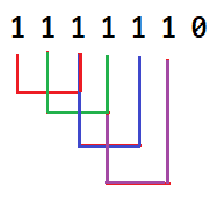# Compute the Count of K Length Subarrays Containing Only 1s in Binary String

## Introduction

Data Structures play a vital role in our problem-solving skills. Consistency with an efficient learning approach can raise your bar in the programming world.

This problem is about counting k length subarrays with only 1’s in the binary(0,1) string.

What do you mean by subarray?

It is a contiguous part of array. For example the array [1, 2, 3, 4].The subarrays are (1), (2), (3), (4), (1,2), (2,3), (3,4), (1,2,3), (2,3,4) and (1,2,3,4).

For questions related to each data structure, refer to the blog Must-Do Coding Interview Questions for Product Based Companies.

Let’s move to our problem statement for better clarity of the problem.

## Problem Statement

We are given a binary string, and we aim to find the count of subarrays of length K that contains only 1.

Let’s understand this with some examples:

### Example1:

Input: s= “1111110” K= 3

Output: 4### Example2:

Input: s= “100001” K= 1

Output: 2

## Approach

First, we will count the size of consecutive ones; after that, we will compute the count of k length subarrays from it.

### Steps

• Add dummy character at last to handle the edge case where the string ends with ‘1’.
• Iterate the binary string from the start.
• Increment the count is ‘1’ is found during iteration.
• As soon as we see ‘0’, store the current count and initialize the count to 0 again.
• Add the count of k length subarray in this group size using relation group size – k + 1.
• Print the final count.

## Implementation

``````import java.util.*;

class Solution{

// To count the k length subarrays
static int count(String s, int k)
{

// If string ends with '1'
s += '0';
int n = s.length();

int c = 0, ret = 0;
for (int i = 0; i < n; i++) {
if (s.charAt(i) == '1')
c++;
else {
if (c >= k) {
ret += (c - k + 1);
}
c = 0;
}
}
return ret;
}

// Main Function
public static void main(String[] args)
{
String str = "1111110";
int K = 3;
System.out.print(count(str, K) +"\n");
}
}``````

## Output

``4``

## Complexities

Time Complexity: The time complexity for this approach is O(n), where n is the length of the binary string.

Space Complexity: O(1) is the space complexity.

## FAQs

1. What is the time complexity to solve this problem?
The time taken by the normal mathematical implementation is O(n), where n is the length of the binary string.

2. What do you mean by subarray?
An array can be divided into subarrays, which are the contiguous part of it.

## Key Takeaways

In this blog, we have covered the following:

• What the problem is, along with the example.
• Approach to ponder upon.
• Implementation in the Java language.

Before stepping into this problem, you can try problems similar to this concept like Arithmetic Subarrays, Subarray Sums I, and many more.

CodeStudio is a one-stop destination for various DSA questions typically asked in interviews to practice more such problems.

Happy Coding!!!